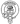# How to use reduce in JavaScript

July 03, 2020

Reduce is one of the key elements of functional programming in JavaScript. It’s an extremely powerful method but also one that can be quite hard to wrap your head around at first glance. One of the first examples we’re going to look at is a classic use case, totaling up values in an array.

``````const numbers = [27, 32, 48, 64, 33]

const sum = numbers.reduce((total, num) => total + num)

console.log(sum)

// 204``````

Let’s take a look at what’s going on under the hood here. We’re looping through the array and calling the reduce function on each item in the array. If we look at this handy table we can see what `total` and `num` are for each step.

step index total num
1 1 27 32
2 2 59 48
3 3 107 64
4 4 171 33
return 204

It might look a little odd that we’re starting from the second item in the array but that’s because if we don’t pass an initial value to our reduce it will use the first value in our array and start from the second. Here’s what our reducer would like if we wanted to pass an initial value:

``````const numbers = [27, 32, 48, 64, 33]

const sum = numbers.reduce((total, num) => total + num, 0)

console.log(sum)

// 204``````

Which would result in a table like this:

step index total num
1 0 0 27
2 1 27 32
3 2 59 48
4 3 107 64
5 4 171 33
return 204

We aren’t limited to using reduce to just total up values. It can be used to flatten arrays:

``````const numberPairs = [
[1, 5],
[3, 7],
[4, 2],
]

const flattened = numberPairs.reduce(
(flat, innerArr) => flat.concat(innerArr),
[]
)

console.log(flattened)

// [1, 5, 3, 7, 4, 2]``````

Count occurrences:

``````const binary = [1, 0, 0, 1, 0, 1]

const occurrences = binary.reduce((occur, num) => {
occur[num] = (occur[num] || 0) + 1
return occur
}, {})

console.log(occurrences)

// {0: 3, 1: 3}``````

Transform an array into an object:

``````const letters = ['a', 'b', 'c', 'd', 'e', 'f']

const lettersObj = letters.reduce((result, item, index) => {
result[index] = item
return result
}, {})

console.log(lettersObj)

// {0: "a", 1: "b", 2: "c", 3: "d", 4: "e", 5: "f"}``````

Or even perform both filter and reduce while only iterating over the array once, say we want to return an array of numbers doubled but only if they are over a certain value:

``````const numbers = [27, 32, 48, 64, 38]

const doubledAbove35 = numbers.reduce((doubled, num) => {
if (num > 35) {
doubled.push(num * 2)
}
return doubled
}, [])

console.log(doubledAbove35)

// [96, 128, 76]``````

Hopefully this article has got the wheels turning in your head and you’ve realised that the only limit when it comes to reduce is your imagination. Just keep in mind though that just because you can do something with reduce doesn’t mean you should.Written by Fraser Hamilton a full stack developer based out of Edinburgh, Scotland.